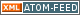www.LinuxHowtos.org howtos, tips&tricks and tutorials for linuxfrom small one page howto to huge articles all in one place

search text in:

Poll
Which kernel version do you use?

poll results

using iotop to find disk usage hogs

using iotop to find disk usage hogs

words:

887

views:

143649

userrating:

average rating: 1.8 (88 votes) (1=very good 6=terrible)

May 25th. 2007:
Words

486

Views

243225

Workaround and fixes for the current Core Dump Handling vulnerability affected kernels

Workaround and fixes for the current Core Dump Handling vulnerability affected kernels

words:

161

views:

126108

userrating:

average rating: 1.3 (28 votes) (1=very good 6=terrible)

April, 26th. 2006:

You are here: manpages

# ATAN2

Section: Linux Programmer's Manual (3)
Updated: 2017-09-15

## NAME

atan2, atan2f, atan2l - arc tangent function of two variables

## SYNOPSIS

```#include <math.h>

double atan2(double y, double x);
float atan2f(float y, float x);
long double atan2l(long double y, long double x);
```

Feature Test Macro Requirements for glibc (see feature_test_macros(7)):

atan2f(), atan2l():

_ISOC99_SOURCE || _POSIX_C_SOURCE >= 200112L
|| /* Since glibc 2.19: */ _DEFAULT_SOURCE
|| /* Glibc versions <= 2.19: */ _BSD_SOURCE || _SVID_SOURCE

## DESCRIPTION

These functions calculate the principal value of the arc tangent of y/x, using the signs of the two arguments to determine the quadrant of the result.

## RETURN VALUE

On success, these functions return the principal value of the arc tangent of y/x in radians; the return value is in the range [-pi, pi].

If y is +0 (-0) and x is less than 0, +pi (-pi) is returned.

If y is +0 (-0) and x is greater than 0, +0 (-0) is returned.

If y is less than 0 and x is +0 or -0, -pi/2 is returned.

If y is greater than 0 and x is +0 or -0, pi/2 is returned.

If either x or y is NaN, a NaN is returned.

If y is +0 (-0) and x is -0, +pi (-pi) is returned.

If y is +0 (-0) and x is +0, +0 (-0) is returned.

If y is a finite value greater (less) than 0, and x is negative infinity, +pi (-pi) is returned.

If y is a finite value greater (less) than 0, and x is positive infinity, +0 (-0) is returned.

If y is positive infinity (negative infinity), and x is finite, pi/2 (-pi/2) is returned.

If y is positive infinity (negative infinity) and x is negative infinity, +3*pi/4 (-3*pi/4) is returned.

If y is positive infinity (negative infinity) and x is positive infinity, +pi/4 (-pi/4) is returned.

## ERRORS

No errors occur.

## ATTRIBUTES

For an explanation of the terms used in this section, see attributes(7).
 Interface Attribute Value atan2(), atan2f(), atan2l() Thread safety MT-Safe

## CONFORMING TO

C99, POSIX.1-2001, POSIX.1-2008.

The variant returning double also conforms to SVr4, 4.3BSD, C89.

acos(3), asin(3), atan(3), carg(3), cos(3), sin(3), tan(3)

## Index

NAME
SYNOPSIS
DESCRIPTION
RETURN VALUE
ERRORS
ATTRIBUTES
CONFORMING TO
COLOPHON||- Powered by- Running on-
Copyright 2004-2020 Sascha Nitsch Unternehmensberatung GmbH::- Level Triple-A Conformance to Web Content Accessibility Guidelines 1.0 -
- Copyright and legal notices -